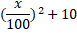# Quiz Discussion

If the arithmetic mean of 0, 5, 4, 3 is a, that of -1, 0, 1, 5, 4, 3 is b and that of 5, 4, 3 is c, then the relation between a, b, and c is

Course Name: Quantitative Aptitude

• 1] a = b = c
• 2] a : b : c = 3 : 2 : 4
• 3] 4a = 5b = c
• 4] a + b + c = 12
##### Solution
No Solution Present Yet

#### Top 5 Similar Quiz - Based On AI&ML

Quiz Recommendation System API Link - https://fresherbell-quiz-api.herokuapp.com/fresherbell_quiz_api

# Quiz
1
Discuss

The average of 11 players of a cricket team is decreased by 2 months when two of them aged 17 years and 20 years are replaced by two new players. The average age of the new players is-

• 1] 17 years 1 month
• 2] 17 years 7 months
• 3] 17 years 11 months
• 4] 18 years 3 months
##### Solution
2
Discuss

Five years ago, the average age of P and Q was 25. The average age of P, Q and R today is 25. Age of R after 5 years will be :

• 1] 15 years
• 2] 20 years
• 3] 40 years
• 4] 35 years
##### Solution
3
Discuss

Nine persons went to a hotel for taking their meals. Eight of them spent Rs. 12 each on their meals and the ninth spent Rs. 8 more than the average expenditure of all the nine. What was the total money spent by them.

• 1] Rs. 115
• 2] Rs. 116
• 3] Rs. 117
• 4] Rs. 118
##### Solution
4
Discuss

Ajay working in a Cellular company as a salesman. His monthly salary is Rs. 200. But he gets bonus as per given rule. If he sells sim cards of Rs. X then his bonus will be. In the first quarter of the year his average sale was Rs. 3000 per month. In the next 5 five month his average sale was Rs. 5000 per month and for next four month his average sale was Rs. 8000 per month. What is the average earning per month for the whole year?

• 1] Rs. 3350
• 2] Rs. 3610
• 3] Rs. 3750
• 4] Rs. 3560
• 5] None of these
##### Solution
5
Discuss

Find the average increase rate, if increase in the population in the first year is 30% and that in the second year is 40%.

• 1] 41%
• 2] 56%
• 3] 40%
• 4] 38%
• 5] 39%
##### Solution
6
Discuss

The average marks of four subjects is 120. If 33 was misread as 13 during the calculation, what will be the correct average?

• 1] 122
• 2] 120
• 3] 125
• 4] 121
##### Solution
7
Discuss

Find the average of the first 40 natural numbers.

• 1]

21

• 2]

20.5

• 3]

21.5

• 4]

None Of These

##### Solution
8
Discuss

The average age of 20 boys in a class is 12 years. 5 new boys are admitted to the class whose average age is 7 years. The average age of all the boys in the class becomes:

• 1] 8.2 years
• 2] 9.5 years
• 3] 12.5 years
• 4] 11 years
##### Solution
9
Discuss

The arithmetic mean of the scores of a group of students in a test was 52. The brightest 20% of them secured a mean score of 80 and the dullest 25% a mean score of 31. The mean score of remaining 55% is-

• 1] 45
• 2] 50
• 3] 51.4 approx
• 4] 54.6 approx
##### Solution
10
Discuss

If the mean of a,b,c is M and ab+bc+ca=0, then the mean of a2,b2,c2 is:

• 1]

M2

• 2]

3M2

• 3]

6M2

• 4]

9M2

# Quiz